Click to Chat

1800-1023-196

+91-120-4616500

CART 0

• 0

MY CART (5)

Use Coupon: CART20 and get 20% off on all online Study Material

ITEM
DETAILS
MRP
DISCOUNT
FINAL PRICE
Total Price: Rs.

There are no items in this cart.
Continue Shopping• Complete JEE Main/Advanced Course and Test Series
• OFFERED PRICE: Rs. 15,900
• View Details

```Revision Notes on Waves and Sound Waves

Waves:-

Wave motion:- Wave motionis the disturbance, set up in the medium, due to the repeated periodic motion of the particles of the medium and travels from the particle to particle, the particles themselves keep vibrating about their mean positions.Wave Equation:- d2y/dt2 = v2 (d2y/dx2)

Transverse wave motion:-It is the type of wave motion in which the particles of the medium are vibrating in a direction at right angles to the direction of propagation of wave.

(a) Velocity of transverse wave,Vt = √T/m = √T/πr2ρ

(b) Vibrations of the particles of medium are normal to the direction of wave propagation.

??Longitudinal wave motion:-It is the type of wave motion in which the particles of the medium vibrate in the direction of propagation of wave.(a) Velocity of longitudinal wave, Vl = √E/ρ

(b) Vibrations of the particles are parallel to the direction of wave propagation.

Terms related to wave motion:-

(a) Wave length(λ):-

(i) It is defined as the distance travelled by a wave during the time particle executing SHM completes one vibration.

(ii) It is the distance between two consecutive particles executing SHM in same phase.

(iii) It is the distance between two consecutive crests or troughs.(b) Wave number (n):- Wave-number of a wave is defined as the reciprocal of wavelength of weave.

n = 1/λ

Unit of weave number is meter.

(c) Velocity of wave:- It is the distance (λ)travelled by the wave during the time (T), a particle completes one vibration.

velocity of wave = (frequency) (wavelength)

v=fλ

(d) Phase:- Phase of a particle is its state which expresses its position and direction of motion.

(e) Phase difference (?):-Phase difference, between two particles, is the difference between their instantaneous phases.

Relation between phase difference (?) and path difference (λ):-

? = (2π/λ)×(path difference)

Simple Harmonic Motion:-A wave which originates from a source, undergoing simple harmonic motion, is called a simple harmonic wave.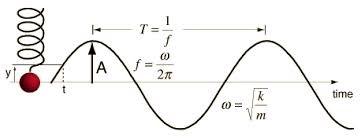Equation:-y=rsinωt

= r sin2πft

= rsin2π(v/λ)t

= r sin2πx/λ

Equation of progressive wave:- A relation between the instantaneous displacement of a particle executing SHM and time is called equation of progressive wave.y = r sin2π (ωt± ?)

y = r sin [(ωt± (2π/λ)x]

y = r sin (ωt± kx)

y = r sin 2π (t/T ± x/λ)

y = r sin 2π/λ (vt± x)

Angular wave number (k): k = 2π/λ

Relation between particle velocity (V) and wave velocity (v):-

V = (2πr/λ) vcos[(2π/λ)(vt±x)]

Vmax = (2πr/λ) v

Energy transmission in a progressive wave:-E = ½ mω2r2

Energy per unit volume:- E = ½ ρr2ω2  Here ρ is the density of medium.

Intensity of a wave:-

I = 2π2ρvf2r2

Intensity of a wave varies directly as the square of its amplitude.

So, I∝r2

Velocity of transverse wave in stretched string:-v = √(T/m), Here,T is the tension in the string.

Interference:- Interference is the phenomenon by virtue of which there is a modification in the distribution of energy due to super position of two or more waves.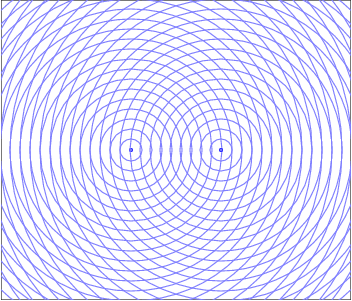y1 = a1sinωt, y2 = a2sin(ωt+?)

y = y1+y2

Amplitude, A = √[a12+a22+2 a1 a2cos?])

Intensity, I = kA2 and I = I1+I2+2(√ I1I2) cos?

Here, I1 = ka12 andI2 = ka22

Angle, θ = tan-1[a2sin?/(a1+a2cos?)]

Constructive interference:-

Phase difference, ? = 2nπ,  n = 0,1,2,3…

A = a1+a2

Imax = [√I1+√I2]2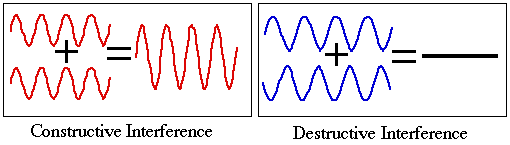Path difference, x = 2n(λ/2)

Destructive interference:-

Phase difference, ? = (2n+1)π,  n = 0,1,2,3…

A = 2acos?/2

I = 4a2k cos2?/2

Imax = 4a2k

Imin = 0

Path difference, x = (2n+1)(λ/2)

Condition for interference:-

(a) The two sources must emit continuous waves having same frequency and wavelength.

(b) The amplitudes of two waves must either be equal or nearly equal.

(c) The two sources should be situated close to each other.

(d) The two sources should be coherent one.

Stationary Wave:-

Wave equation, y = 2acos (2π/λ) x sin (2π/λ) vt

Amplitude, A = 2acos(2π/λ) x

Condition for maxima (anti-nodes), x = k(λ/2)

Condition for minima (nodes), x = (2k+1)(λ/4)

Frequency of transverse vibrations in stretched string:-

f=(1/2l) √(T/m), Here l is the length, T is the tension and m is the mass.

f=(1/lD) √(T/πρ), Here l is the length, T is the tension, D is the diameter and ρ is the density.

Harmonics in stretched strings:-

(a)  First harmonic (fundamental frequency),  f0=(1/2l) √(T/m)

(b)  Second harmonic (first overtone), f1 = 2f0=(2/2l) √(T/m)

(c)  Third harmonic (second overtone), f2 = 3f0=(3/2l) √(T/m)

(d) pth harmonic (p-1 overtone),  fp-1 = pf0=(p/2l) √(T/m)

Here, p=1,2,3…

Frequency of tuning fork:-

f∝(t/l )√(E/ρ)

Here, t is the thickness, l is the length, E is the elastic constant and ρ is the density.

Phenomenon of Beats:-Periodicvariations of amplituderesulting from the superposition of two waves of slightly different frequencies is known as phenomenon of beats.

If m is the number of beats per second, then, m = f1-f2. Here f1 and f2 are the frequencies of the two waves.

y1 = asin2πf1t, y2 = asin2πf2t

y = y1+y2= Asin2πft

Amplitude, A = 2acos2π(f1-f2/2)t, Frequency, F = f1-f2/2

(a) Maxima:- t = f/f1-f2

(b) Minima:- t = 2f+1/2(f1-f2)

Beat period (tb):- It is defined as the time interval between consecutive beats or it is the time between two consecutive maxima or minima of intensity of sound.

tb = 1/f1-f2

If m is the number of beats per second, then,

m = 1/beat period = f1-f2

This signifies, the number of beats per second is equal to the difference in frequencies of two waves.

Sound Waves:-

It is the form of energy which produces, in us, the sensation of hearing.

Properties:-

(a) Longitudinal in nature.

(b) It requires a material medium for its propagation.

(c) Sound waves can be reflected.

(d) Sound waves suffer refraction.

(e) Sound waves show the phenomenon of interference

(f) Sound waves shows diffraction

(g) Sound propagates with a velocity much smaller than that of light.

(h) Sound gets absorbed in the medium through which it passes.

Loudness (L)or Intensity (I):-

L∝logI

So, L = K log10(I1/I0)

Unit of intensity of sound is bel.

Intensity (I) and Amplitude (A):- I∝A2

Intensity(I) and distance from the source (r):- I∝1/r2

Pitch or Shrillness:-Pitch is a sensation which determines the shrillness of sound. It is subjective and cannot be measured quantitatively. It depends up on frequency and relative motion between the sources and the listener.

Quality or Timber:- It is that characteristic of a musical sound which enables us to distinguish between two notes of the same pitch and loudness produced  by two different sources.

Velocity u of longitudinal wave (sound) [Newton’s Formula]:-

u =√E/ρ

Here E is the coefficient of elasticity and ρ is the density of medium.

Velocity of sound in solids:-

u =√Y/ρ

Here Y is the young’s modulus of elasticity and ρ is the density.

Velocity of sound in liquids:-

u =√B/ρ

Here B is the Bulk modulus of elasticity and ρ is the density.

Velocity of sound in gases:-

u=√γP/ρ

Here, γ (=cP/cV) is the adiabatic ratio, P is the pressure and ρ is the density.

Various factors affecting velocity of sound:-

(a) Effect of density:- The velocity of sound in a gas varies inversely as the square root of its density. u1/ u2 = √[ρ2/ρ1]

(b) Effect of moisture:-um/ud= √[ρd/ ρm]

Since, ρm<ρd, then, um>ud

This signifies sound travels faster in moist air.

(c) Effect of pressure:- u=√γP/ρ=√γk = constant

This signifies, change of pressure has no effect on the velocity of sound.

(d) Effect of temperature:- ut/u0 =√ρ0/ρt= √T/T0

Thus, velocity of sound varies directly as the square root temperature on Kelvin’s scale.

(e) Temperature coefficient of velocity of sound (α):- α = u0/546 = (ut-u0)/t

Overtones in open pipe:-An open pipeis openat both ends. Since air is free to vibrate at an open end, we must get an antinode at the open end.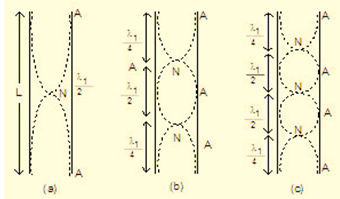(a) Fundamental frequency:-

Wavelength,  λ=2l

Frequency,  f=u/2l = (1/2l)√(γP/ρ)

Here l is the length of the pipe and u is the velocity of sound.

(b) First overtone (Second Harmonic):-

Wavelength,  λ1=l

Frequency,  f1=2f

(c) Second overtone (Third Harmonic):-

Wavelength,  λ2=2l/3

Wavelength,  f2=3f

Overtones in closed pipe:-Since air, at a closed end, is not free to vibrate, there must be a node at a closed end always.(a) Fundamental frequency:-

Wavelength,  λ=4l

Frequency,  F=u/4l = (1/4l)√(γP/ρ)

Here l is the length of the pipe and u is the velocity of sound.

(b) First overtone (Third Harmonic):-

Wavelength,  λ1=(4/3)l

Frequency,  F1=3F

(c) Second overtone (Fifth Harmonic):-

λ2=4l/5

F2=5F

Comparison of fundamental frequencies of a closed end of an open pipe:- f = 2F

Doppler’s Effect:-Theapparent change in pitch of a note, due to the relative motion between the source and the listener is called Doppler’s effect.

(a) Source in motion, listener at rest:-

(i)  Source approaching the listener:-

Modifying wave length, λ' = V-a/f

Apparent frequency, f' = [V/V-a]f

Change in frequency, ?f = (a/V-a)f

Here V is the velocity of sound in air and a is the velocity of source when it moves towards the listener.

(ii) Source going away from the listener:-

Apparent frequency,f' = [V/V+a]f

Change in frequency, ?f = -(a/V+a)f

(iii) Source crossing the listener:-

Apparent frequency of the source before crossing = (V/V-a) f

Apparent frequency of the source after crossing = (V/V+a) f

Change in frequency, ?f = -(2aV/V2-a2)f

(b) Source at rest, listener in motion:-

(i)  Listener moving away from source:-

Apparent frequency,f'= [V-b/V]f

Change in frequency, ?f = (-b/V)f

Here b is the velocity of listener.

(ii) Listener moving towards the source:-

Apparent frequency:-f'= [V+b/V]f

Change in frequency, ?f = (+b/V)f

(iii) Listener crossing the source:-

Apparent frequency of the source before crossing = (V+b/V) f

Apparent frequency of the source after crossing = (V-b/V) f

Change in frequency, ?f =-2fb/V

(c) Source and listener both in the medium:- Change in frequency due to relative motion of source and listener.

Source (S)#

Listener (L) (X)

Nature of velocities

Expression for f '

#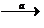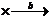+ve, +ve

f ' = (V-b/V-a) f

#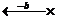+ve, -ve

f ' = (V+b/V-a) f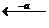#-ve, -ve

f ' = (V+b/V+a) f#-ve, +ve

f ' = (V-b/V+a) f

(d) Effect of motion of medium:-

Apparent frequency:-f ' = [(R-b)/(R-a)] f

Here, R = V+ωcosθ, ω is the velocity of wind, θ is the angle between direction of propagation of sound and that of wind.

```### Course Features

• 728 Video Lectures
• Revision Notes
• Previous Year Papers
• Mind Map
• Study Planner
• NCERT Solutions
• Discussion Forum
• Test paper with Video Solution